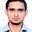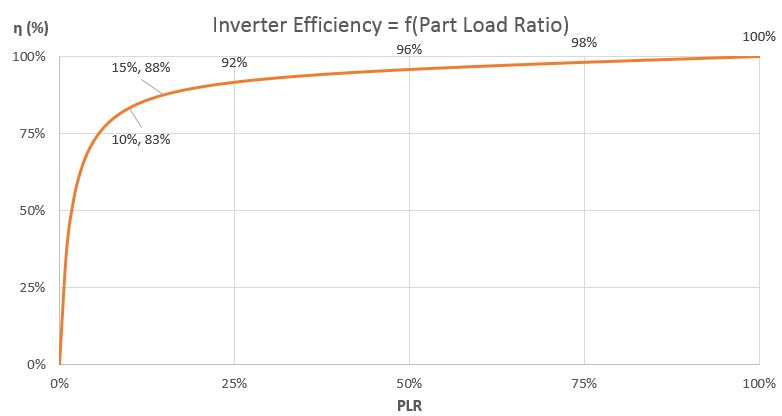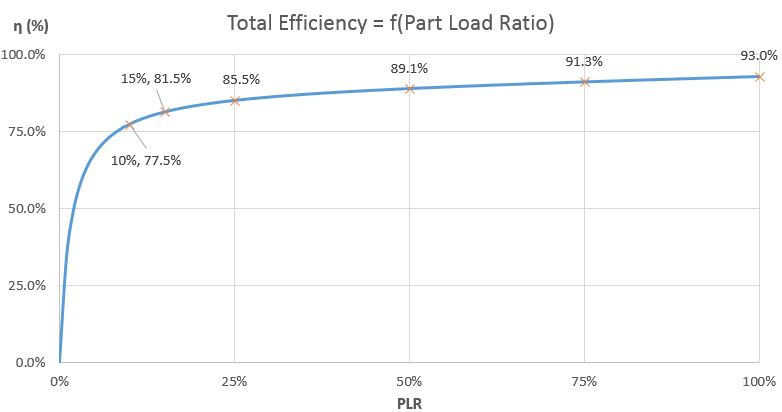Question-and-Answer Resource for the Building Energy Modeling Community
Get started with the Help page

# what percentage of battery loss and other losses are counted in eQUEST for modelling of SPV plant ? (please reply with source)I am modelling a Solar PV plant on eQUEST, I need to know various losses this software considers for calculation of outputs and where would I get part load efficiency curve of inverter which is used as default?

edit retag close merge delete

Sort by » oldest newest most voted

Ashok,

You're a little out of luck as far as getting proper sources for this, at least in terms for something clearly written and directly quotable. I've looked into the DOE-2 Engineers Manual version 2.1A (here) and there is nothing about photovoltaic generators. I suppose it was added later, after 1993.

Though, looking at eQuest and the help files, you can already understand what is going on. I've looked at the source code of DOE22 as well, especially in PLT.LIS that is in the folder SRC48r, and you could do the same.

Anyway, in DOE2 you'll enter the characteristics of your PV Modules, especially the performance data. That's basically going to play on the efficiency of your modules. Then, you'll create your generator. A number of parameters come into play in the calculation routine. The array tilt for example is used to determine the electric capacity (direct and diffuse solar, angle of incidence, air mass, and ambient temperature are the parameters for that).

As far as modeling the "other losses", what you really want to be looking at is the EIR and the EIR-FPLR curves:

ELEC-INPUT-RATIO

specifies the ratio of direct-current input power to alternating-current output power. This number is the inverse of the full-load efficiency, and as such is always greater than 1.0.

EIR-FPLR

accepts the U-name of a curve that modifies the nominal electric input ratio as a function of the hourly part load ratio. This use of this curve is identical to part-load curves documented elsewhere.

I guess you might also play with the auxiliary power, but I think the main goal of this was to include say the power used for a sun tracking system.

Choose the ELEC-INPUT-RATIO to include all your regular losses such as wiring losses, potential step up transformer losses, etc. Choose the EIR-FPLR to match your inverter characteristics.

The EIR-FPLR that is included by default in eQuest is called "Inverter EIR-fPLR" and it's easy to see its coefficients. For your convenience, it's a Quadratic curve (Z = a + bX + cX²) with coefficients (a, b, c) = (0.01677774, 1.03766644, -0.05444432).

Now, this is not what you are used to see. What you are used to see is the efficiency of the inverter. The default eQuest inverter looks like this:If I assume an ELEC-INPUT-RATIO of 1.075 (= 93% efficient, I'm voluntarily taking a fairly low number in order to be able to see the difference), the Total Efficiency of the system would look like:I hope this clarifies things.

more

hey Julien many thanks for response, (At 50% load) Z = a + bX + cX², Z = 0.01677774 + 1.03766644 * 0.5 - 0.05444432 * 0.5 * 0.5 = 0.522, Is this okay ?. According to curve, the value of Z should be 0.96

@Ashok Dhayal: Correct, EIR(PLR=50%) = 0.522. The curve is the efficiency... that's not the same. I got Actual EIR by multiplying EIR with full-load EIR I chose (1.075*0.522=0.561) and then Total efficiency by doing PLR/Actual EIR=89.1%

In particular for Solar PV the industry is advancing so quickly that a set of default values made at some unknown time in the past may not be accurate for a modern PV system.

If it is possible to substitute your own performance from a current product that might be the preferred path.

more